# Geometry - math problems

#### Number of problems found: 1473

• Mike chose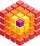Mike chose 4 identical cubes, 3 identical prisms and 2 identical cylinders from the kit. The edge of the cube is 3 cm long. The prism has two dimensions the same as the cube, its third dimension is 2 times longer. The diameter of the base of the cylinder
• Draw a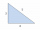Draw a triangle ABC, if you know: alpha = 60° side b = 4 cm side a = 10 cm
• MIT 1869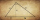You know the length of hypotenuse parts 9 and 16, at which the hypotenuse of a right triangle is divided by a perpendicular running from its opposite vertex. The task is to find the lengths of the sides of the triangle and the length of the line x. This a
• Edges of the cuboid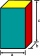Find the length of the edges of the cuboid, which has the following dimensions: width is 0.4 m; the height is 5.8 dm and the block can hold 81.2 liters of fluid.
• The surfaceThe surface of the cylinder is 1570 cm2, its height is 15 cm. Find its volume and radius of the base.
• What power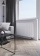What power should the radiator have in a room 5x4 meters with a height of 3 meters? Hint: Calculate 35 watts per meter ^ 3 in case you need 22 degrees in the room but you need about 24 degrees in the bathroom. It's an old heating cheat, but it will defini
• A butterA butter cube with an edge 6.5 cm long is packed in a package with dimensions a = 28 cm, b = 15 cm. Calculate how many cm2 the package is larger than the surface of the cube.
• Dana helped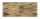Dana helped her dad build a sandbox for her younger sister. The sandbox is shaped like a rectangular prism that is 4 1/2 feet long and 4 feet wide. Dana used bags of sand to fill the sandbox 1/2 of a foot deep. Each bag contained 1/2 of a cubic foot of sa
• Length of the chord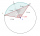Calculate the length of the chord in a circle with a radius of 25 cm with a central angle of 26°.
• The conical roof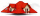The conical roof above the warehouse has a diameter of the lower part (base) d = 11.2 m and a height v = 3.3 m. How many rectangular steel plates with dimensions of 1.4 m and 0.9 m were needed for the production of this roof, if the seams and waste requir
• School modelThe beech school model of a regular quadrilateral pyramid has a base 20 cm long and 24 cm high. Calculate a) the surface of the pyramid in square decimeters, b) the mass of the pyramid in kilograms if the density of the beech is ρ = 0,8 g/cm ^ 3
• Water cylinderZuzana poured 785 ml of water into a measuring cylinder with a base radius of 5 cm. The water in the cylinder reached a height of 2 cm from the upper edge of the cylinder. How tall is the cylinder? (π = 3.14)
• An experiment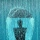The three friends agreed to the experiment. At the same time, they all took out an empty cylindrical container on the windowsill and placed it so that it was horizontal. Everyone lives in a different village, and each used a container with a different bot
• Waste container eco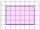The waste box has the shape of a block with dimensions of 1.8 m, 1.5 m, 1.2 m. 0.25 m3 of waste is added to it every day. How many days will it be filled?
• Right-angled triangle baseFind the volume and surface area of a triangular prism with a right-angled triangle base if the length of the prism base legs are 7.2 cm and 4.7 cm and the height of a prism is 24 cm.
• How toHow to find a total surface of a rectangular pyramid if each face is to be 8 dm high and the base is 10 dm by 6 dm.
• Empty aquariumHow much does an empty aquarium weigh with dimensions: length = 40 cm, width = 30 cm, height = 20 cm, if 1 dm2 of glass weighs 300 g? Calculate its weight in kilograms.
• Cylinder container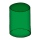If the cylinder-shaped container is filled with water to a height of 5 dm, it contains 62.8 hectoliters of water. Calculate the diameter of the bottom of the container. Use the value π = 3.14.
• Frustrum - volume, areaCalculate the surface and volume of the truncated cone, the radius of the smaller figure is 4 cm, the height of the cone is 4 cm and the side of the truncated cone is 5 cm.
• Mr. Gardener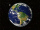Mr. Gardener wants to make wood for the balcony. Boxes. Each will have the shape of a perpendicular prism with a square base, the height is limited to 60 cm. Each container will be filled with soil by pouring the whole bag of substrate sold in a package w

Do you have an exciting math question or word problem that you can't solve? Ask a question or post a math problem, and we can try to solve it.

We will send a solution to your e-mail address. Solved examples are also published here. Please enter the e-mail correctly and check whether you don't have a full mailbox.

Please do not submit problems from current active competitions such as Mathematical Olympiad, correspondence seminars etc...